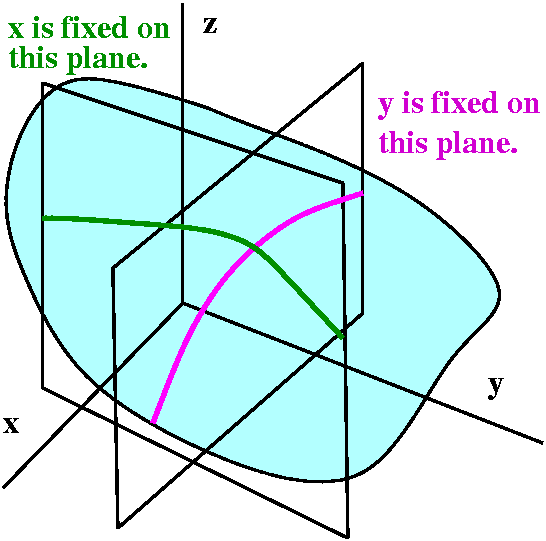prerequisite
1.derivative (only one variable)

(1)it measures the sensitivity of f(x) with respect to a trivial change of x (slope of the tangent line)(from wiki)
2. partial derivative (multi variable, but not any direction)
at lease two variables.
Actually,it’s derivative based on each dimension(x bar,y bar ,z bar….)

(2)3.directional derivative (multi variables ,any direction)
break a vector down  into each dimension,so we can use partial derivative to solve thisactually it’s just vector dot product ([partial derivative] * [vector in this direction])
it’s all about how the vector in this direction affects the partial derivative.we only care about the direction of the vector,so vector v is a unit vector

gradient is about finding a direction which we can get the steepest slope. it means we need to find a direction which maximize [partial derivative ]* [initial vector].
multiplication of two vectors ,obviously when they stick together(theta = 0) ,we can get the steepest slope. meanwhile ,we only care about the direction.
so the gradient = [partial derivative]

In conclusion, if we want to minimize the cost function, we can decrease each direction in the vector by its gradient respectively. This is gradient descent.

another way to comprehend.if we want to minimize the cost , we should findto makenegative.
suppose we choose, alpha is a small,positive parameter.
thenso the cost will be negative.this is what we looking for.
when, we can minimize the cost function

## catalan numbers

Posted on Categories math

## 三角函數Posted on Categories math